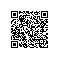# 从0打卡leetcode之day 6--最长回文串

### 题目描述

输入: "babad"

输入: "cbbd"



### 解题

#### 优化策略

    public String longestPalindrome(String s) {

//先判断是否为空或者长度小于1
//把||写成了&&害我找了好久都不知道错在哪...
if(s == null || s.length() < 1){
return "";
}
int left = 0;//用来记录子串的起始位置
int right = 0;//用来记录子串的末尾位置

for(int i = 0; i < s.length(); i++){
//通过findMore这个方法来拓展
//bab这种情况
int t1 = findMore(s, i, i);//bab这种情况
//abba这种情况
int t2 = findMore(s, i, i+1);

//选出比较长的那个
int max = Math.max(t1, t2);
if(max > right - left){
left = i - (max - 1)/2;
right = i + max/2;
}
}
return s.substring(left, right+1);
}

public int findMore(String s, int left, int right){
while(left >= 0 && right < s.length()
&& s.charAt(left) == s.charAt(right)){
left--;
right++;
}
return right - left - 1;
}使用钉钉扫一扫加入圈子
+ 订阅